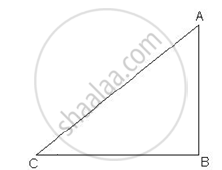Share

# In the below given figure, a tower AB is 20 m high and BC, its shadow on the ground, is 20√3 m long. Find the sun’s altitude. - CBSE Class 10 - Mathematics

ConceptIntroduction to Trigonometry Examples and Solutions

#### Question

In the below given figure, a tower AB is 20 m high and BC, its shadow on the ground, is 20√3 m long. Find the sun’s altitude.#### Solution

LetAB be the tower and BC be its shadow.
AB = 20, BC = 20√3
In ΔABC,

tan theta=

tan theta=20/(20sqrt3)

tan theta=1/sqrt3

but,tan30=1/sqrt3

theta=30^@

The Sun is at an altitude of 30º .

Is there an error in this question or solution?

#### Video TutorialsVIEW ALL 

Solution In the below given figure, a tower AB is 20 m high and BC, its shadow on the ground, is 20√3 m long. Find the sun’s altitude. Concept: Introduction to Trigonometry Examples and Solutions.
S